# KSEEB Solutions for Class 9 Maths Chapter 13 Surface Areas and Volumes Ex 13.8

In this chapter, we provide KSEEB SSLC Class 9 Maths Chapter 13 Surface Areas and Volumes Ex 13.8 for English medium students, Which will very helpful for every student in their exams. Students can download the latest KSEEB SSLC Class 9 Maths Chapter 13 Surface Areas and Volumes Ex 13.8 pdf, free KSEEB SSLC Class 9 Maths Chapter 13 Surface Areas and Volumes Ex 13.8 pdf download. Now you will get step by step solution to each question.

## Karnataka Board Class 9 Maths Chapter 13 Surface Areas and Volumes Ex 13.8

(Assume π = 227, unless stated otherwise)

Question 1.
Find the volume of a sphere whose radius is
(i) 7 cm
(ii) 0.63 m
Solution:
(i) r = 7 cm, V = ?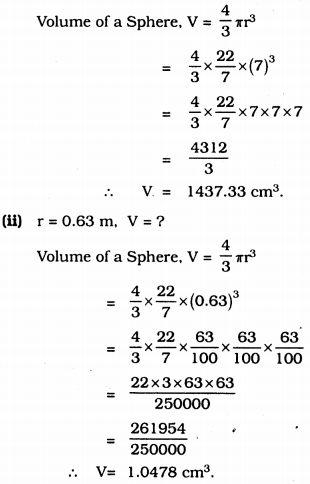Question 2.
Find the amount of water displaced by a solid spherical ball of diameter
(i) 28 cm.
(ii) 0.21 m.
Solution:
(i) Diameter of solid spherical ball, diameter, d = 28 cm,
∴ radius, r = 282 = 14 cm.
Volume of solid spherical ball, V = 43 πr3.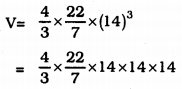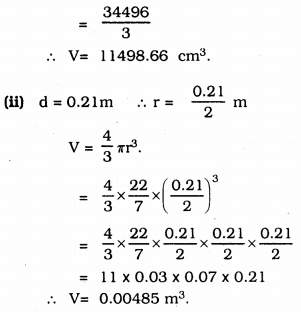Amount of water displaced by the ball = 0.00485 m3.

Question 3.
The diameter of a metallic ball is 4.2 cm. What is the mass of the ball, if the density of the metal is 8.9 g per cm3 ?
Solution:
Diameter of metallic ball, d = 4.2 cm. density = 8.9 gm/cm3
mass, m = ? d = 4.2 cm.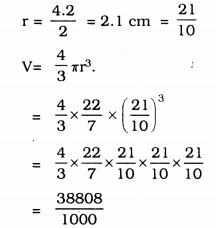∴ V = 38.808 cm3.
∴ Mass = Density × Volume
= 8.9 × 38.808
= 345.40 gm.

Question 4.
The diameter of the moon is approximately one-fourth of the diameter of the earth. What fraction of the volume of the earth is the volume of the moon?
Solution:
Let the diameter of earth be ‘d’ unit.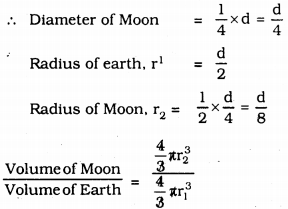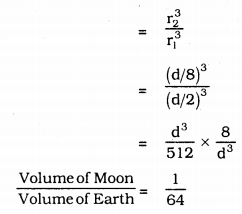∴ Volume of Moon = 164 × Volume of Earth

Question 5.
How many litres of milk can a hemispherical bowl of diameter 10.5 cm hold?
Solution:
Diameter of a hemispherical bowl, d = 10.5 cm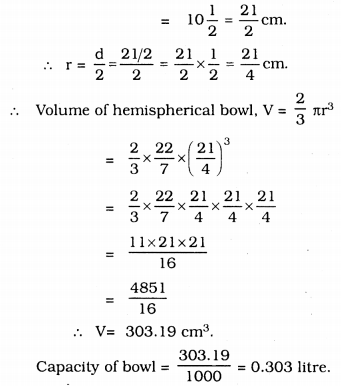Question 6.
A hemispherical tank is made up of an iron sheet 1 cm thick. If the inner radius is 1 m, then find the volume of the iron used to make the tank.
Solution:
In a hemispherical tank,
Inner radius, r1 = 1 m
Outer radius, r2 = 1 + 0.01 = 1.01 m (∵ 1 cm = 0.01)
∴ Volume of hemispherical tank, V
= Volume of outer diameter – Volume of inner diameter.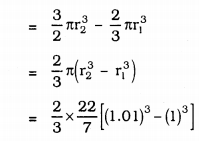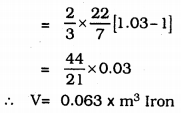Question 7.
Find the volume of sphere whose surface area is 154 cm2.
Solution:
Surface area of sphere, 4πr2 =154 cm2.
Volume of Sphere, V = ?
A = 4πr2 = 154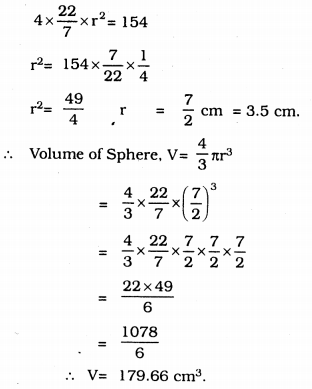Question 8.
A dome of a building is in the form of a hemisphere. From inside, it was white-washed at the cost of Rs. 498.96. If the cost of white-washing is Rs. 2.00 per square metre, find the
(i) inside surface area of the dome,
(ii) volume of the air inside the dome.
Solution:
(i) Cost of white-washing dome is Rs. 498.96
Cost of white-washing is Rs. 2 per sq. metre.
∴ Surface Area = 498.962 = 249.48 m2.

(ii) Surface Area of hemisphere, V = 2πr2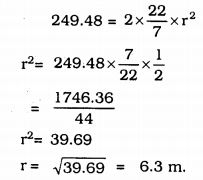∴ Inner volume of hemisphere dome, V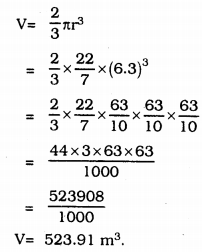Question 9.
Twenty seven solid iron spheres, each of radius r and surface area S are melted to form a sphere with surface area S1. Find the
(i) radius r of the new sphere,
(ii) ratio of S and S1
Solution:
(i) Volume of 1 sphere, V = 43πr3
Volume of 27 solid sphere
= 27 × 43πr3
Let r1is the radius of the new sphere.
Volume of new sphere = Volume of 27 solid sphere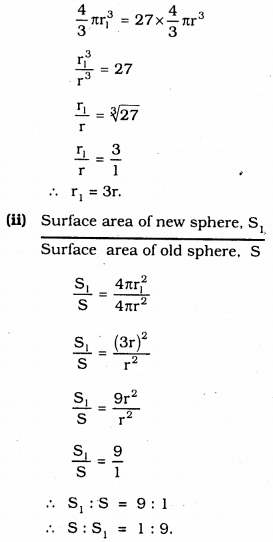Question 10.
A capsule of medicine is in the shape of a sphere of diameter 3.5 mm. How much medicine (in mm3) is needed to fill this capsule?
Solution:
Diameter of capsule of medicine, d
d = 3.5 mm = 72 mm.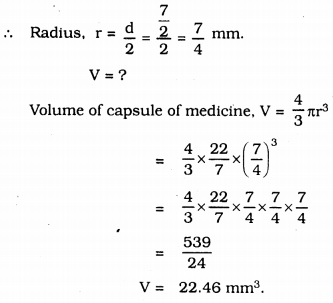All Chapter KSEEB Solutions For Class 9 Maths

—————————————————————————–

All Subject KSEEB Solutions For Class 9

*************************************************

I think you got complete solutions for this chapter. If You have any queries regarding this chapter, please comment on the below section our subject teacher will answer you. We tried our best to give complete solutions so you got good marks in your exam.

If these solutions have helped you, you can also share kseebsolutionsfor.com to your friends.

Best of Luck!!